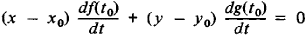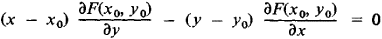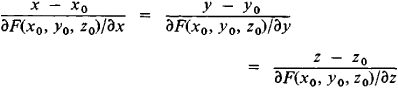# normal

(redirected from Normal (disambiguation))
Also found in: Dictionary, Thesaurus, Medical.

## Normal,

town (1990 pop. 40,023), McLean co., central Ill.; inc. 1865. It is the center of a productive farming region. Motor vehicles are manufactured in Normal. The town originally grew around Illinois State Univ. (1857; formerly called Illinois State Normal Univ.), which remains a major contributor to its economy.
The following article is from The Great Soviet Encyclopedia (1979). It might be outdated or ideologically biased.

## Normal

(to a curve or surface). The normal at a given point of a curve or surface is a line that passes through this point and is perpendicular to the tangent line or tangent plane at the same point of the curve or surface. A plane curve has at each point a single normal that lies in the plane of the curve. If x = f(t) and y = g(t) are parametric equations of a plane curve L, then the equation of the normal at point (x0, y0) of curve L that corresponds to the value to of the parameter; may be written asFor a plane curve defined by the equation F(x,y) = 0, the equation of the normal has the formA space curve has at each point an infinite set of normals, and these normals all lie in a certain plane (the normal plane). The normal that lies in the osculating plane is called the principal normal. The normal that is perpendicular to the osculating plane is called the binormal. The tangent, the principal normal, and the binormal form the moving trihedral of the curve.

For the surface defined by the equation F(x, y, z) = 0, the normal can be represented by the equationsThe concept of the normal plays a significant role not only in differential geometry but also in various applications of this subject, such as geometrical optics and mechanics. The formulation of the fundamental laws of refraction and reflection of light rays is an example from geometrical optics; in mechanics, a mass point or a physical body experiences a reaction directed along the normal when it is displaced along smooth curves or surfaces; in a conservative field, the lines of force at each point are directed along the normal to the isopotential surface that passes through the point.

## normal

[′nȯr·məl]
(meteorology)
The average value of a meteorological element over any fixed period of years that is recognized as standard for the country and element concerned.
McGraw-Hill Dictionary of Scientific & Technical Terms, 6E, Copyright © 2003 by The McGraw-Hill Companies, Inc.

## normal

i. As it pertains to meteorology, the value of an element averaged for a given location over a period of time, normally fifty years, and recognized as a standard.
ii. Equivalent to usual, regular, rational, or standard conditions.
iii. At a right angle to the datum under reference.

## normal

1. Psychol
a. being within certain limits of intelligence, educational success or ability, etc.
b. conforming to the conventions of one's group
2. Biology Med (of laboratory animals) maintained in a natural state for purposes of comparison with animals treated with drugs, etc.
3. Chem (of a solution) containing a number of grams equal to the equivalent weight of the solute in each litre of solvent.
4. Chem denoting a straight-chain hydrocarbon: a normal alkane. Prefix: n-, e.g. n-octane
5. Geometry another word for perpendicular
6. Geometry a line or plane perpendicular to another line or plane or to the tangent of a curved line or plane at the point of contact
Collins Discovery Encyclopedia, 1st edition © HarperCollins Publishers 2005
Site: Follow: Share:
Open / Close